Next: 4.5 Interface to a Up: 4. RF-Extraction Previous: 4.3 Application Example

# 4.4 Extracted Device Quantities: Invariants

The well known definition for the current gain cut-off frequency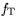is given in (4.10).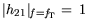(4.10)

This definition ofhas various approximations in terms of intrinsic small-signal equivalent elements. The most important approximations for a HEMT are (assuming the parasitic inductances and capacitances to be stripped off) according to Baeyens in :(4.11)

and even simpler in (4.12) neglecting the parasitic resistances and the output conductance: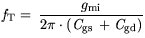(4.12)

On the contrary, the inclusion of the pad capacities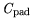and the fringe part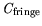from (4.4) in (4.11) yield an extrinsic charging time and extrinsic, respectively.(4.13)

The intrinsic transconductance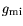and the extrinsic transconductance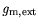are then related by: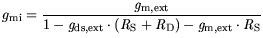(4.14)

where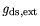is the extrinsic output conductance. Using (4.11)-(4.13)is precisely defined for different levels of deembedding.

The quantity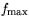is defined in several manners depending on the invariants used for its definition . Defining the Unilateral Power Gain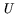allows for the highest values ofin a device representing the maximum gain in a lossless reciprocal deembedding .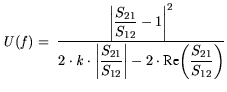(4.15)

Second, the Maximum Available Gain (MAG) and the Maximum Stable Gain (MSG) can be used for defining: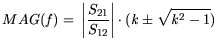(4.16)

for the stability factor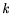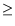1.is then determined as: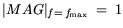(4.17)

The MAG drops with a slope of -20 dB/dec as a function of frequency near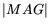=1. Kurokawa's stability factoris defined from the S-parameters as :(4.18)

The Maximum Stable Gain (MSG) is used for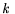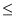1 is defined as: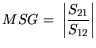(4.19)

The MSG drops with -10 dB/dec as a function of frequency. The transition between the two for= 1 defines the frequency, from whichbased on MAG/MSG eventually can be extrapolated with a slope of -20 dB/dec for a given gate width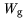. The frequency: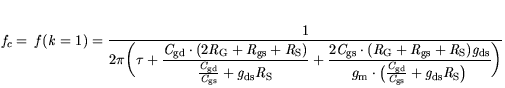(4.20)

defines the stability point and is expressed as a function of small-signal elements .depends the single finger gate width and is a critical quantity for amplifier design, especially for mm-wave applications. It will be analyzed in Chapter 7 with respect to statistical changes of mm-wave devices.Next: 4.5 Interface to a Up: 4. RF-Extraction Previous: 4.3 Application Example
Quay
2001-12-21